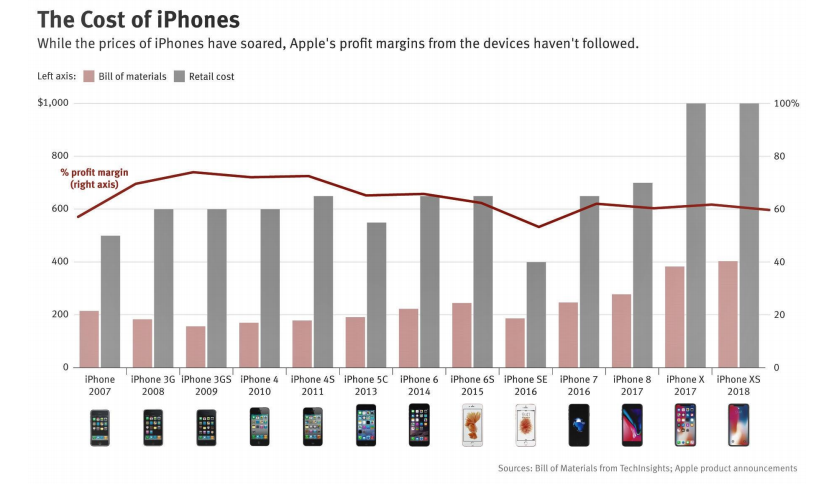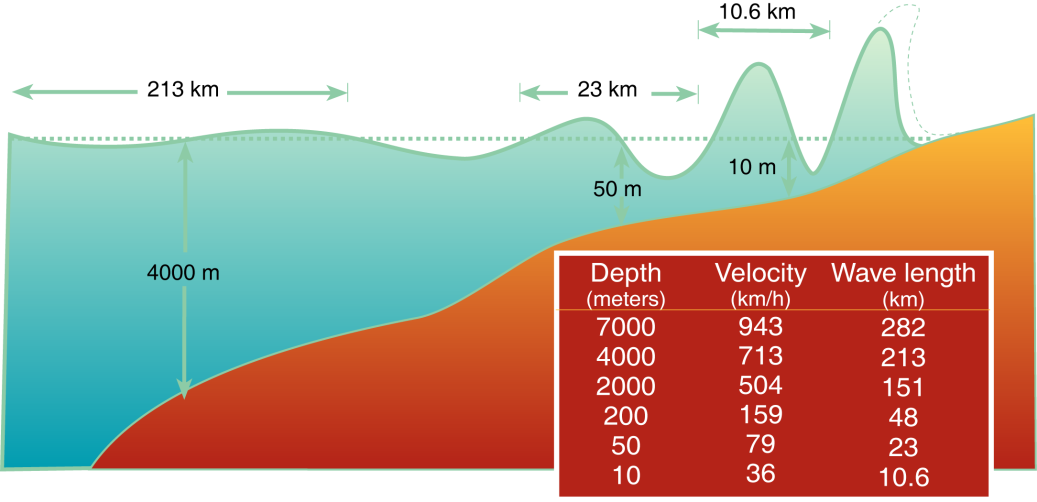# FRESHMAN SCIENCE SUMMER PACKET

All science classes utilize math which is why your child(ren) need(s) to be abreast with the material listed on the next few pages. The attached packet is for Freshman science students to complete over the summer and bring with them on the first day of class to their science class to be assessed on completion. All work is to be completed on loose leaf. Final answers for each calculation problem should be boxed. Graphing problems must be completed on graph paper. This packet will be reviewed during the first few weeks of school. Since each lesson in the course contains some type of mathematical reference, this packet will be referred to during the entire school year. We advise all students to secure this packet.

Each section of the packet is clearly headed, followed by instructions, and a Khan Academy video explaining how to solve each problem or expression. For further clarification, you can create a Khan Academy account at www.khanacademy.org/math to view endless math equations, problems, and expressions. A Khan Academy account is not necessary to access the attached links.

Parents, thank you in advance for assuring your child(ren) will complete this packet before school begins. Students, we look forward to seeing you in September.

Have a great summer,

Freshman Science/Math Summer Packet

Basic Multiplying & Dividing. Evaluate.

1. (−2)(−4) =      2.)  15÷3 =                 3.)    8(−1) =                  4.)   (-21)÷(-7) =

5.) 26(−12) =     6.)   (-300)÷6 =         7.)  (−73)1 =               8.)   (-72)÷(-9) =

9.)    7(3) =                     10.)    0÷(-20) =

Percents. Read the word problem then solve to find the correct percentage.

12.)  The art club had an election to select a president. 9 out of the 12 members of the art club voted in the election. What percentage of the members voted?

13.)  A school assembly had 30 students in attendance, and 20% of them were first-graders. How many first-graders were at the assembly?

14.)   Brenda's Diner sold 10 milkshakes last week. 40% of the milkshakes had whipped cream on top. How many milkshakes with whipped cream were sold?

15.)  At the sewing store, Ava bought a bag of mixed buttons. She got 21 buttons in all. 21 of the buttons were large. What percentage of the buttons were large?

16.)  Ben earns \$12,800 a year. About 15% is taken out for taxes. How much is taken out for taxes?

17.)  What percentage of 80 is 50?                            18.)   20 is what percentage of 25?

19.)   What is 60% of 0?                                             20.)   Find 10% of the number 50.

21.) 6 + (-12) + (-2) =                         22.)  3 - (-13) =                        23.) ______ * (-8) = 32

24.) (-190) ÷ 2 =                                 25.)  (-10) ÷ ___ = 5                   26.) (-16) - (-27) =

27.) __ * (-9) = (-54)                          28.)   (-60) ÷ (-12) =               29.)   8 + 15 + 14 =

30.)   (-5) - 8 =                                    31.)    (-5) * 5 =                        32.)    (-4) * (-9)=

Exponents. Evaluate each expression- Show all work

33.) 10 3 =                  34.)  10-3 =             35.)  (1/ 2 ) 5 =         36.) 109 =         37.)  10 =

Write the following expressions using exponents.

38.)   45 * 45 * 45 * 45 =

39.) (−0.7) * (−0.7) * (−0.7) * (−0.7) * (−0.7) * (−0.7) * (−0.7) * (−0.7) =

Evaluate.

40.)   104 + 012 =                                                           41.)   26 ÷ 42 =

42.)   07 − 115 =                                                    43.) 93 ÷ 18 =

Measurement Conversions.  Convert.- Show all work

44.) 37 cm = _______mm                  45.)  20 m = _______cm           46.)  34 m = _______ mm

47.) 20 m = _______ km                    48.)  29 km = _______ m            49.)  36 km = ______ cm

50.) 100 g = _______ kg                    51.)  24 kg = _______ g             52.)  8.3 g = _______ kg

53.)  The metric unit of measurement for mass is ___________________________.

54.)  The metric unit of measurement for weight is __________________________.

55.)  The decimal equivalent for a meter is ________________________________.

56.)  The decimal equivalent for a centimeter is ___________________________.

57.)  When you move the decimal point two places to the left to convert a metric unit, it is the

same as ______________ the measurement by 100.

58.)  When you move the decimal point two places to the right to convert a metric unit, it is the

same as ______________ the measurement by 100.

Scientific Notation. Convert the following numbers into scientific notation.

59.) 3,400 = _______                  60.)  0.000023 = _______                      61.)  4.50 =_______

62.) 1,000,000 = _______           63.)  0.00671= _______

Convert the following numbers into standard notation.

64.) 2.30 * 104 = _______      65.)  1.76 * 103= _______          66.) 1.901 * 10-7=_______

67.) 1.76 * 100 = _______           68.)  5.40 * 101= _______

Fractions (adding and subtracting, multiplying and dividing). Evaluate each.

69.) 6/12 + 2/10=            70.)  4/8 + 3/4 =             71.)  1/2 * 2/5 =                   72.) 1¼* 3=

73.) 1/4 ÷ 9/10 =              74.)  8/10 ÷ 2/5 =           75.)  12/25 - 11/25 =         76.)  1⅜- ⅞ =

Order of Operations. Evaluate each.

Remember, PEMDAS (Please Excuse My Dear Aunt Sally) stands for: Parentheses Exponents Multiplication Division Addition Subtraction

77.)  14 +18 ÷ 2 * 18 =                                                     78.)  15 * 18 + 12 ÷ 3 + 9 =

79.) (11 + 42 – 5) ÷ ( 11 – 4 ) =                                         80.)  (10 + 59 – 32) ÷ (24 – 4) =

Graphical Analysis of Scientific Data

Graphing Data and Analysis Questions for Graph of the Week

Using the attached graph/data complete the following questionsQuestions.

1. What is the title of the Graph? _______________________________________

1. What scales are used on the graph?__________________ Why?___________________________________________________________

1. What units are being utilized in the graph?________________

Why? ________________________________________________________

1. What quantities are being compared?__________________________________

1. Are there x and y axises?____________

If so, What does the x-axis represent?_______________________________

What does the y-axis represent?____________________________________

How does that relate to the independent and dependent Variables?

__________________________________________________________________

1. What type of graph is this?___________________________

1. Is there an upward or downward trend?___________

Are there any sudden spikes in the graph?________

If so, what accounts for them?________________________________________ If not why would they not be there? ____________________________________

1. What do you foresee happening in the future?__________________________ What prediction can you make for the future? __________________________ ____________________________________________________

1. What inferences can you make about the graphs? ______________________

_____________________________________________________________

1. What are the limitations of the graphs? _______________________________

_______________________________________________________________

1. Is this data accurate?______________________

Do we believe this information is true?_______________________________ Why or Why not? ________________________________________________

1. Is this data meaningful? ______________________________

Do we believe this information is important?___________

Why or Why not?_________________________________________________

1. What choices did the person who created these graphs make? _________________________________________________

Why did they make those choices? ___________________________________

_______________________________________________________________

1. For each graph: Identify any changes, trends or differences you see in the graph or figure. ________________________________________________________

________________________________________________________________

Write 3 “What I see” comments based on what you have identified.

1) ________________________________

2) ________________________________

3)_________________________________

For each “What I see” comment written, interpret the meaning by writing a “what it means” comment. ____________________________________________________

______________________________________________________________________________________________________________________________________

1. How are the two graphs related?___________________________________ _____________________________________________________________

What information do they provide together that individually they do not?_________________________________________________________

Which choice most accurately represents the information in the graph?

1.  The difference between the average daily high and the average daily low is constant throughout the year
2. The difference between the average daily high and the average daily low is greatest on June 10
3. The difference between the average daily high and the average daily low is lowest on March 5
4. The difference between the average daily high and the average daily low is the same on both June 10 and Sep 8

The loss of ice due to melting of the ice sheet would be expected to be least on

1.  March 5
2. Jun 10
3. Sep 8
4. Dec 13

Reading Passage:  Tsunami speed as a function of ocean depth.

A tsunami is a very long-wavelength wave of water that is generated by sudden displacement of the seafloor or disruption of any body of standing water.  Tsunami are sometimes called "seismic sea waves", although they can be generated by mechanisms other than earthquakes.  Tsunami have also been called "tidal waves", but this term should not be used because they are not in any way related to the tides of the Earth.  Because tsunami occur suddenly, often without warning, they are extremely dangerous to coastal communities.

Wave velocity is the speed of the wave. Velocities of normal ocean waves are about 90 km/hr while tsunami have velocities up to 950 km/hr (about as fast as jet airplanes), and thus move much more rapidly across ocean basins

The table below shows depth and velocity data for a tsunami.  From this information:

1. Define dependent variable ________________________________________ _________________________________________________________________

1. Define independent variable ________________________________________

__________________________________________________________________

1. Identify the dependent variable. _______________________________________

1. Identify the independent variable _____________________________________

1. On graph paper, create a velocity-depth graph from the information in the data table.

1. State the type of graph that is characteristic of velocity with depth? ______________________________

Data:

 Depth (meters) Velocity (km/h) Wavelength (km) 7000 943 282 4000 713 213 2000 504 151 200 159 48 50 79 23 10 36 10.6
1. Predict the tsunami speed for the following ocean depths:
2. 8000 meters  __________________________
3. 3500 meters ___________________________
4. 70 meters _____________________________
5. 5 meters _______________________________

The diagram below includes the wavelength of a tsunami.

1. On graph paper, create a graph of the wavelength with ocean depth.
2. What type of graph is characteristic of wavelength with depth? _________________________The following questions 81-94 are for students who have already completed Algebra I.

Factoring and Distributing. Simplify by factoring or distributing.

81.) 2(x + 3) =                  82.) 2(x + 3 + y) =           83.)  −5 (2x − 3) =

84.)  –5(–8w + p) =          85.) 20 + 32w =     86.)  84 + 36z =

87.)   (2x - 6)(5x + 7) =                                            88.)  (y - 10)(4y + 2) =

Solving One Step Equations.  Solve each word problem.

A linear equation is of the form y=mx+b

Use the distance formula to solve questions 89-92; d = vt, where d is the distance traveled, v is the velocity and t is the time.

89.)  A car travels at 40 km/hr for 2 hours and at 55 km/hr for 2 hours.  How far has the car traveled? What is its average velocity?

90.)  How long will it take an airplane to travel 1,250 kilometers if it is traveling at 150 km/hr?

91.)  A car is traveling at 5 m/s.  How far has it gone in 12 seconds?

92.)  A train travels 600 kilometers in 1 hour.  What is the train’s velocity in meters/second?

93.)  There were 32 students in Jaden’s class eating lunch. Then, more students joined Jaden’s class. Now there are 86 total students eating lunch. How many students joined Jaden’s class?

94.)  Kari, Katelynn, and Morgan went out for dinner and split the bill evenly. The total bill was \$46.68. How much did each pay?